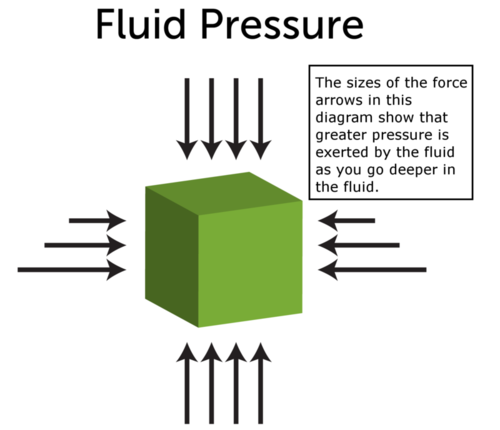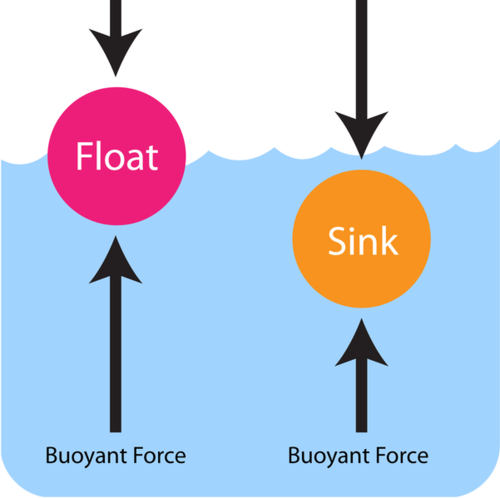Buoyancy Force

Big Ideas

• Buoyant force is an upward force that fluids exert on any object that is placed in them. Buoyant force occurs because the fluid below an object exerts greater pressure on the object than the fluid above it.
• If an object’s weight is less than the buoyant force acting on it, then the object floats. If an object’s weight is greater than the buoyant force acting on it, then the object sinks.
• A given volume of a denser substance is heavier than the same volume of a less dense substance. Therefore, density of an object also affects whether it sinks or floats.

What Is Buoyant Force?

Buoyant force is an upward force that fluids exerts on any object that is placed in them. The ability of fluids to exert this force is called buoyancy. What explains buoyant force? A fluid exerts pressure in all directions, but the pressure is greater at greater depth. Therefore, the fluid below an object, where the fluid is deeper, exerts greater pressure on the object than the fluid above it. You can see in the Figure below how this works. Buoyant force explains why the girl pictured above can float in water.Q: You’ve probably noticed that some things don’t float in water. For example, if you drop a stone in water, it will sink to the bottom rather than floating. If buoyant force applies to all objects in fluids, why do some objects sink instead of float?

A: The answer has to do with their weight.

Weight and Buoyant Force

Weight is a measure of the force of gravity pulling down on an object, whereas buoyant force pushes up on an object. Which force is greater determines whether an object sinks or floats. Look at the Figure below. On the left, the object’s weight is less than the buoyant force acting on it, so the object floats. On the right, the object’s weight is greater than the buoyant force acting on it, so the object sinks.Because of buoyant force, objects seem lighter in water. You may have noticed this when you went swimming and could easily pick up a friend or sibling under the water. Some of the person’s weight was countered by the buoyant force of the water.

Density and Buoyant Force

Density, or the amount of mass in a given volume, is also related to the ability of an object to float. That’s because density affects weight. A given volume of a denser substance is heavier than the same volume of a less dense substance. For example, ice is less dense than liquid water.